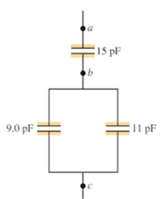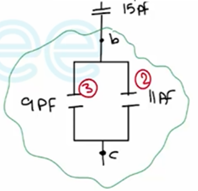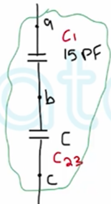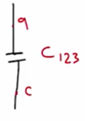Need Help?

Subscribe to Physics 2

###### \${selected_topic_name}
• Notes

$\begin{array}{l}{\text { For the system of }} \\ {\text { capacitors shown in Fig. E2 4.16, }} \\ {\text { find the equivalent capacitance }} \\ {\text { (a) between } b \text { and } c, \text { and }(b)} \\ {\text { between } a \text { and } c .}\end{array}$(1) $C_{e q}=C_{23}=c_{2+C_{3}}$

$=11+9=20 \mathrm{pF}$

(2) $\frac{1}{c_{123}}=\frac{1}{c_{1}}+\frac{1}{c_{23}}$

$=C_{123}=C_{eq}=\frac{C_{1} C_{23}}{C_{1}+C_{23}}$$∴\quad=\frac{15+20}{15+20}=8.6 \mathrm{PF}$